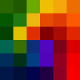We are moving our forum in GitHub Discussions. For questions about Phalcon v3/v4 you can visit here and for Phalcon v5 here.

Greetings!

I'm trying to pixelate a image via GD library using the following code

``````\$images = '../uploads/';

\$image->pixelate(50);
\$image->save(\$images. 'output.jpg');``````

and I'm getting a weird result where only certain areas are being pixelated.

Is this a bug or am I doing something wrong?4.0k

No. I think it's not Bug. Try use smaller `pixelate` paramteter

``\$image->pixelate(2);``2.2k

It's doing this with any value I throw at it, always leaving same non-rendered pixel square in between. Since (2) is really tiny to see it, here's one with a value of (10).

No. I think it's not Bug. Try use smaller `pixalate` paramteter

``\$image->pixelate(2);``4.0k
Accepted

Ok. I understand what do you mean now :) Can you try this:

``````<?php

{
protected function _pixelate(\$amount)
{
\$x = 0;
while (\$x < \$this->_width) {
\$y = 0;
while (\$y < \$this->_height) {
\$x1 = \$x + \$amount/2;
\$y1 = \$y + \$amount/2;
\$color = imagecolorat(\$this->_image, \$x1, \$y1);

\$x2 = \$x + \$amount;
\$y2 = \$y + \$amount;
imagefilledrectangle(\$this->_image, \$x, \$y, \$x2, \$y2, \$color);

\$y += \$amount;
}
\$x += \$amount;
}
}
}``````

and then

``````\$images = '../uploads/';

\$image = new GdExtendPixelate(\$images. 'input.jpg');
\$image->pixelate(10); //or 5, or 10, or ....
\$image->save(\$images. 'output.jpg');``````4.0k2.2k

Perfect! Thank you so much.

Ok. I understand what do you mean now :) Can you try this:

``````<?php

{
protected function _pixelate(\$amount)
{
\$x = 0;
while (\$x < \$this->_width) {
\$y = 0;
while (\$y < \$this->_height) {
\$x1 = \$x + \$amount/2;
\$y1 = \$y + \$amount/2;
\$color = imagecolorat(\$this->_image, \$x1, \$y1);

\$x2 = \$x + \$amount;
\$y2 = \$y + \$amount;
imagefilledrectangle(\$this->_image, \$x, \$y, \$x2, \$y2, \$color);

\$y += \$amount;
}
\$x += \$amount;
}
}
}``````

and then

``````\$images = '../uploads/';

\$image = new GdExtendPixelate(\$images. 'input.jpg');
\$image->pixelate(10); //or 5, or 10, or ....
\$image->save(\$images. 'output.jpg');``````